Measurement of Particle Size Distribution in Turbid Solutions by Dynamic Light Scattering Microscopy

Chemistry

Summary

A protocol for the direct measurement of particle size distribution in concentrated solutions using dynamic light scattering microscopy is presented.

Hiroi, T., Shibayama, M. Measurement of Particle Size Distribution in Turbid Solutions by Dynamic Light Scattering Microscopy. J. Vis. Exp. (119), e54885, doi:10.3791/54885 (2017).

Abstract

A protocol for measuring polydispersity of concentrated polymer solutions using dynamic light scattering is described. Dynamic light scattering is a technique used to measure the size distribution of polymer solutions or colloidal particles. Although this technique is widely used for the assessment of polymer solutions, it is difficult to measure the particle size in concentrated solutions due to the multiple scattering effect or strong light absorption. Therefore, the concentrated solutions should be diluted before measurement. Implementation of the confocal optical component in a dynamic light scattering microscope1 helps to overcome this barrier. Using such a microscopic system, both transparent and turbid systems can be analyzed under the same experimental setup without a dilution. As a representative example, a size distribution measurement of a temperature-responsive polymer solution was performed. The sizes of the polymer chains in an aqueous solution were several tens of nanometers at a temperature below the lower critical solution temperature (LCST). In contrast, the sizes increased to more than 1.0 µm when above the LCST. This result is consistent with the observation that the solution turned turbid above the LCST.

Introduction

Particle size is one of the most fundamental properties of colloidal and polymer solutions. Numerous techniques are used to measure the particle size. Particle sizes of 1.0 µm or larger can be measured directly using an optical microscope. For smaller particles, alternative techniques, such as laser diffraction, electron microscopy, or atomic force microscopy, are used2,3. Dynamic light scattering is a commonly-used technique for the measurement of particle size distributions in solutions4. The results obtained using this technique are not derived from images of the particles but from the characteristic time of the fluctuations in scattered light intensity. These fluctuations originate from Brownian motion, which is characterized by a diffusion constant. The size distribution is obtained from the distribution of diffusion constants using the Einstein-Stokes equation. Due to its simplicity, dynamic light scattering is widely used for the routine assessment of solutions, such as paints and food colloids.

Pretreatment is required for most of the techniques used for the particle size measurement of solution samples. In the case of electron microscopy and atomic force microscopy, the sample must be analyzed under vacuum conditions. Therefore, it is difficult to observe the samples in their native forms. Furthermore, for laser diffraction and dynamic light scattering, only diluted samples that are free from multiple scattering and light absorption can be measured. To overcome this difficulty, several new techniques have been proposed for the measurement of dynamic light scattering from undiluted, concentrated solutions, such as cross-correlation spectroscopy5,6, low-coherence dynamic light scattering7,8, diffusing-wave spectroscopy9,10, and differential dynamic microscopy11,12.

We have developed a new apparatus called a dynamic light scattering microscope1. This apparatus enables us to measure turbid samples without dilution by means of a confocal optical system in which multiple scattering is eliminated using a pinhole. However, the measurement procedure and data analysis are slightly more complicated than those of commercially-available instruments. This video explains the measurement procedure and data analysis in detail using the analysis of the temperature-responsive polymer, poly(N-isopropylacrylamide), as an example.

Protocol

1. Sample Preparation

1. Purification of temperature-responsive monomers
1. Dissolve 20 g of N-isopropylacrylamide (NIPA) in 100 mL of toluene.
2. Filter the solution under suction to eliminate dust.
3. Mix the filtrate with 500 mL of petroleum ether.
4. Place the reaction vessel in an ice-water bath.
5. Stir the solution until the monomers are precipitated (typically 30 min).
6. Filter the solution under suction to obtain the precipitated monomers.
7. Dry the monomers under reduced pressure (100 Pa) overnight.
2. Preparation of the temperature-responsive polymer solution
1. Degas 20 mL of deionized water for 1.0 min using a diaphragm pump.
2. Dissolve 780.8 mg of the purified NIPA in 9.5 mL of degassed and deionized water.
3. Place the reaction vessel in an ice-water bath.
4. Shield the reaction from light by covering the apparatus with aluminum foil.
5. Stir the solution gently for 10 min while introducing a moderate flow of Ar gas gently via a pipette tip attached to the gas cylinder with a tube.
6. Add 11.9 µL of N,N,N',N'-tetramethylethylenediamine to the solution via a micropipette.
7. Stir the solution for 1.0 min while introducing Ar gas, as mentioned in step 1.2.5.
8. While stirring the sample, dissolve 4.0 mg of ammonium persulfate in 0.5 mL of degassed and deionized water.
9. Mix the sample solution (from step 1.2.7) and ammonium persulfate solution (from step 1.2.8).
10. Stir the solution for 30 s while introducing Ar gas, as mentioned in step 1.2.5.
11. Cover the solution with aluminum foil and keep it in a refrigerator (4 °C) overnight.
3. Preparation of sample mounts
1. Place 60 µL of the sample solution (from step 1.2.11) in a cavity slide.
2. Cover the solution with a circular cover glass. Be careful not to trap air bubbles.
3. Remove excess solution using a micropipette and laboratory wipes.
4. Seal the sample with glue. Let the glue dry at room temperature (typically 6 h).
5. Prepare another slide filled with 0.1 wt% polystyrene latex (100-nm particle diameter) suspension by following steps 1.3.1-1.3.4. This slide is used as a standard.

2. Particle Size Measurement with a Dynamic Light Scattering Microscope

1. Optimization of the instrument
1. Place the polystyrene latex suspension slide (from step 1.3.5) on the stage of the inverted microscope. The cover glass side should face downward.
2. Place a beam damper in front of the detector (an avalanche photodiode and an autocorrelator).
3. Apply a laser beam (solid-state laser, λ = 488 nm, 30 mW, continuous wave) to the sample through an objective lens (10×). A portion of the reflected light goes through a launch mirror of the microscope and is observed by a CCD camera mounted at the side port of the microscope (Figure 1).
4. Adjust the height of objective lens to set the focal point at the sample suspension by shifting the height of objective lens from the low-to-high position. During this procedure, the reflected image is focused three times: at the surface of the cover glass, at the interface between the cover glass and the sample, and at the interface between the sample and the hole-slide glass. Set the focal point between the second and third points.
5. Attenuate the scattered light intensity by changing the laser power.
6. Introduce the scattered light into the detector by removing the beam damper in front of the detector. This unit measures the time correlation of the light intensity.
7. Set a pinhole (ϕ = 50 µm) between the microscope and the detector to achieve the confocal effect. Adjust the position of the pinhole to maximize the light intensity at the detector.
8. Measure the time correlation function of scattered light intensity for 30 s by initiating the operation of the correlator via a computer. The measured correlation function is often expressed as g(2) (t) - 1, where t is the correlation time4 and. Here, I(t) is the scattered light intensity at the time t and (•••)T is time averaging. The decay time will be approximately 0.1 ms.
9. Adjust the focal point to obtain a wide range for the initial amplitude of the time correlation function (g(2) (t = 0) - 1).
NOTE: The initial amplitude is strongly affected by the amount of reflected light. By moving the focal point toward the interface between the cover glass and the sample, the amount of reflected light increases. For strong light scatterers, such as polystyrene latex, the initial amplitude can be altered from 0 to 1. However, it is difficult to set the initial amplitude close to 1 for more common polymer solutions because the intensity of the reflected light is much higher than that of the scattered light.
10. Apply the inverse Laplace transformation (using the constrained regularization program CONTIN13,14) to the obtained time correlation function to acquire the size distribution function. In cases where the initial amplitude is set to less than 0.2, the distribution function of the hydrodynamic radius will show a sharp peak around 100 nm, which is twice the actual radius (see the discussion for details).
2. Sample measurement
1. Set the stage temperature to 25 °C.
2. Place a slide prepared with poly-NIPA (PNIPA) solution (step 1.3.4) on the stage of the microscope.
3. Measure the time correlation function of the scattered light intensity by following steps 2.1.4-2.1.8. If the initial amplitude is larger than 0.2, adjust the focal point to make the initial amplitude of the time correlation function less than 0.2 by following step 2.1.9. A small initial amplitude simplifies the analysis.
4. Set the stage temperature to 35 °C and wait until the solution turns turbid. The lower critical solution temperature (LCST) of PNIPA solution is 32 °C15.
5. Measure the time correlation function by following steps 2.1.4-2.1.8. If feasible, adjust the position of the focal point to make the initial amplitude of the time correlation function less than 0.2. For turbid solutions, their initial amplitudes tend to increase, since the intensity of the scattered light increases while that of the reflected light remains constant.
6. Apply the inverse Laplace transformation to the obtained time correlation functions to obtain the size distribution functions. Note that the actual size is half the obtained value in cases where the initial amplitude is less than 0.2.

Representative Results

Time correlation functions of scattered light intensity for a polystyrene latex suspension (particle radius: 50 nm) were measured at different focal points, as shown in Figure 2(a). These correlation functions were converted into the distribution functions of the hydrodynamic radius by the inverse Laplace transformation (refer to Figure 2(b) and (c)). Using the same procedure, the time correlation functions and distribution functions of the hydrodynamic radius of the PNIPA solution were obtained at 25 °C and 35 °C, respectively. Figures 3(a) and (b) show the time correlation functions of the scattered light intensity and the corresponding size distribution functions of the PNIPA solution below (25 °C) and above (35 °C) the LCST. The size distribution functions were obtained by the inverse Laplace transformation followed by the correction of the partial heterodyne. The average hydrodynamic radius below the LCST is several tens of nanometers, which is typical for polymer solutions. In contrast, the hydrodynamic radius above the LCST is about 1.0 µm. This result is consistent with the fact that the solution is turbid above the LCST. The red and blue lines in Figure 3 represent the size distribution of PNIPA solutions obtained immediately after and 20 min after the solution became turbid, respectively. Figure 3(b) clearly indicates the growth of the aggregation.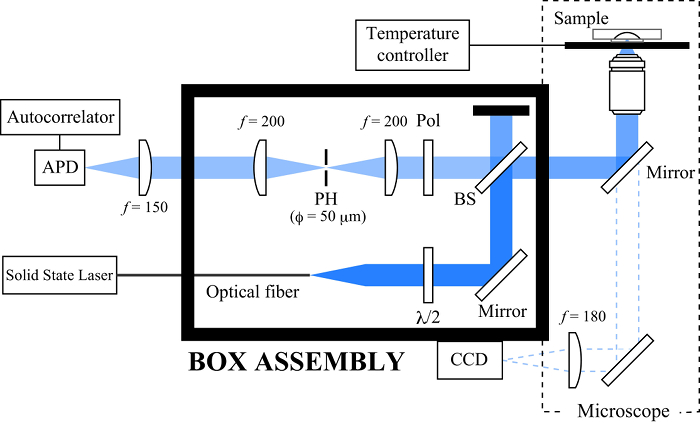Figure 1. Schematics of the dynamic light scattering microscope. Pinhole (PH), beam splitter (BS), polarizer (Pol), and avalanche photodiode (APD). Please click here to view a larger version of this figure.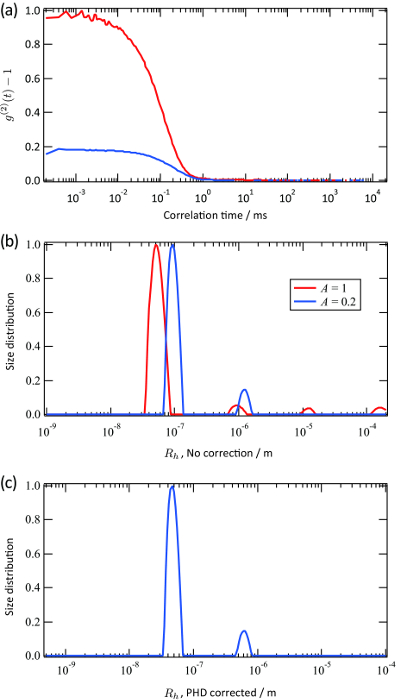Figure 2. Representative results for a polystyrene latex suspension. (a) Time correlation functions of the scattered light intensity for the polystyrene latex suspension. The nominal radius is 50 nm and the concentration is 0.1 wt%. Two datasets were obtained from different scattering points. (b), (c) Corresponding size distribution functions for the polystyrene latex suspension obtained by the inverse Laplace transformation of (a). The red line corresponds to the time correlation function whose initial amplitude is approximately 1.0, and the blue line corresponds to that with an initial amplitude that is approximately 0.2. The horizontal axis was calculated without (b) and with (c) considering the effect of partial heterodyning (PHD) when A << 1. Please click here to view a larger version of this figure.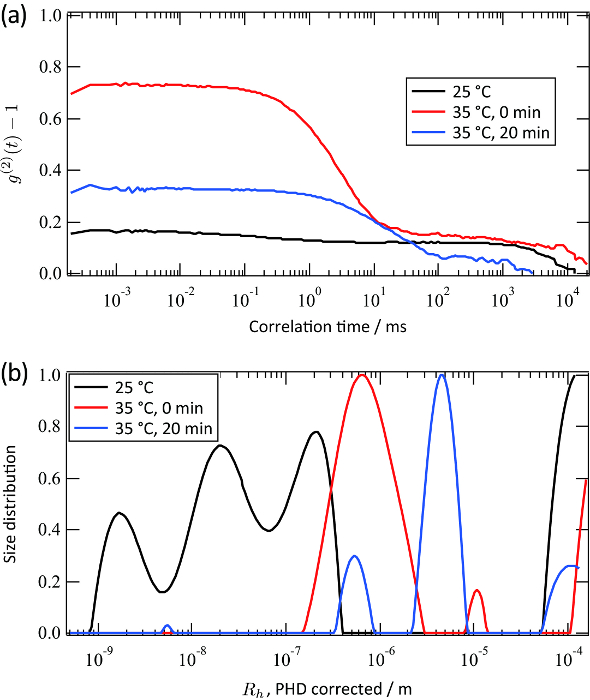Figure 3. Representative results for a PNIPA solution. (a) Time correlation functions of scattered light intensity for the PNIPA solution. (b) Corresponding size distribution functions for the PNIPA solution obtained by the inverse Laplace transformation of (a). The horizontal axis was calculated considering the effect of partial heterodyning for each data set. The black line represents the data obtained at 25 °C. The red line represents the data obtained just after the solution turned turbid (35 °C). The blue line represents the data obtained after a 20-min measurement of the red line. Please click here to view a larger version of this figure.

Discussion

The initial amplitude of the time correlation function heavily depends on the focal point, as shown in Figure 2(a). This seemingly contradicts the fact that the solution is homogeneous (except for the thin layer at the interface)8. This variation in the initial amplitude is attributed to a variation in the amount of reflected light. Partial heterodyne theory16 predicts that the initial amplitude, A, the scattered light Intensity, Is, and the reflected light intensity, Ir, satisfy the following equation1This equation shows that the larger Ir becomes, the smaller A becomes. Therefore, A is reduced by setting the focal position close to the interface. The apparent diffusion constant DA can be obtained by fitting the time correlation function in the case of monodisperse solutions: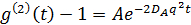where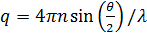. Here, n is the refractive index of the solvent (water, 1.33), θ is the scattered angle (180°), and λ is the wavelength of light (514.5 nm). Since we applied backscattering geometry, the value of q is fixed. However, this point is solved by using different wavelengths of light. Please note that any kind of continuous-wave laser source is available to construct the DLS microscope. Thanks to the small irradiated volume, the coherence factor17 is estimated to be more than 0.99 and is negligible. For polydisperse solutions, the distribution function of DA is obtained by the inverse Laplace transformation. Partial heterodyne theory also predicts that DA is not the same as the actual diffusion constant D. These two diffusion constants satisfy the following equation:The diffusion constant D is converted into the hydrodynamic radius Rh using the Einstein-Stokes equation4. When A = 1, this relationship becomes DA = D. In this case, the data conversion process is the same as that for the common dynamic light scattering. The red line shown in Figure 2(b) corresponds to this case. In contrast, this relationship becomes DA = 0.5D at the limit of A → 0. Therefore, the size is estimated to be twice as large as the actual size when A is small (practically, less than 0.2), as shown by the blue line of Figure 2(b). If we know that A is significantly small, the horizontal axis can be shifted, as shown in Figure 2(c). In principle, we can convert DA into D for any value of A. In practice, however, it is better to set the initial amplitude smaller than 0.2, since the simple approximation DA ∼ 0.5D holds true.

The prominent features of the dynamic light scattering microscope technique were demonstrated using a PNIPA solution. The conformation of PNIPA below and above the LCST has been extensively studied using small-angle neutron scattering15,18. In contrast, dynamic light scattering has not been utilized for the analysis of PNIPA above the LCST because of its turbidity19. This problem is solved by the dynamic light scattering microscope, as shown in Figures 3(a) and (b). The size of these aggregates is several µm, which cannot be obtained by either small-angle X-ray/neutron scattering or conventional light scattering techniques. Time-resolved measurements using this system give information on the aggregation process during the temperature change.

The drawback of the dynamic light scattering microscope is also illustrated in Figure 3. For the result below the LCST, the time correlation function is strongly affected by the very small amount of dust present (the black lines in Figure 3). For example, the time correlation function does not decay completely, even with correlation times in the order of 1.0 s. This is because the volume irradiated with this apparatus (approximately 1.0 µm) is significantly smaller than that irradiated with the usual dynamic light scattering apparatus (approximately 100 µm). In cases where the intensity of scattered light is weak, the signal is obscured by the noise, such as that caused by small amounts of dust in the solution. Therefore, the three peaks shown in Figure 3(b) may not have quantitative importance although the general order of the size is meaningful. Note that such a weak scatterer can be measured by a conventional dynamic light scattering apparatus.

We have demonstrated that the dynamic light scattering microscope enables us to measure both transparent and turbid samples with the same setup. Since the optical path length in the samples is short, this technique can be applied to strong light-absorbing samples, such as carbon nanotube suspensions20. In addition, due to its high spatial resolution, this technique can be applied to biological cells. For its application to biology, this method can also be combined with other imaging techniques, such as fluorescence and Raman imaging. Thus, we believe that the dynamic light scattering microscope is a powerful tool for a wide range of research fields.

Disclosures

The authors have nothing to disclose.

Acknowledgments

This work has been financially supported by Grants-in-Aid for Scientific Research from the Ministry of Education, Culture, Sports, Science, and Technology (No. 25248027 to M.S.).

Materials

 Name Company Catalog Number Comments N-isopropylacrylamide, 98% Tokyo Chemical Industry Co., Ltd. I0401 toluene, 99% Wako Pure Chemical Industries, Ltd. 201-01876 petroleum ether, distillation temperature 30 ~ 60 °C Wako Pure Chemical Industries, Ltd. 169-22565 N,N,N',N'-tetramethylethylenediamine, 99% Sigma T9281 ammonium persulfate, 98% Sigma 248614 polystyrene latex suspension, 1 wt% Duke Scientific Corporation 3500A argon Koike Sanso Kogyo Co., Ltd. purity > 99.999 vol.% cavity slide Matsunami Glass Ind.,Ltd. 83-0336 inverted microscope Nikon Instech Co., Ltd. ECLIPSE Ti-U Thermo Plate Tokai Hit CO.,Ltd TP-108R-C Solid-state laser Coherent OBIS 488LX avalanche photodiode ALV-GmbH ALV-High Q.E. Avalanche Photo Diode correlator ALV-GmbH ALV-5000/EPP